# Convert negative values to positive and positive to negative

Hi all. I’m running a “Date&Time Difference” node to essentially create a column that counts from a particular date in time. Everything from that date to now is negative (-1, -50, -80) and everything from before that date is positive (1, 100, 450). I know doing a math formula with abs() will turn them all into positive values. What is the best way to convert the negative values to positive and the positive values to negative. I know via the “Date&Time Difference” node I can just reverse the order in which the dates are being subtracted, but is there a rule engine or way via the nodes to do this as well?

Thanks!

Would `-1 * \$your_column\$` using the math node do the job ?

Hope it helps

Best Ael

6 Likes

One could go even shorter and use `-\$yourColumn\$` instead!8 Likes

Hi @tdewagner & welcome to the KNIME community

I guess the difficulty here comes from the list of proposed functions in the -Math Formula- node which does not include the basic mathematical operators “+,-,*,/”.

The -Math Formula- node allows writing a mathematical formula as a combination of functions and basic mathematical operations. Parenthesis “(,)” to prioritize operations are also allowed. As explained in the help, one can also deal with NaN values (or missing values) with the following operators:

``````NaN values act as value true in boolean expressions and
compared to anything (including NaN)
in any way (including ==, but not !=,
which is like !(... == ...)) is false (0).
``````

Moreover, there is an “if-then-else” condition which allows writing conditional mathematical operations, for instance:

`if( \$value\$ >=0, \$value\$, 0)`

Here the formula is for instance setting to zero any negative value. Another example here:

In the precise case of needing to change the sign of a number, @Thyme’s solution is definitely the most appropriate.

Hope all these explanations help.

Best

Ael

2 Likes

Just for the sake of correctness, it does it actually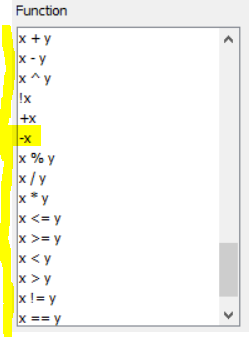Best

Ael

3 Likes

Good one @Thyme , I was not aware of this. I would have done the * -1 as suggested by @aworker.

3 Likes

Thanks all for the advice. Somehow in the midst of trying to learn KNIME, I forgot the basics of multiplication (*-1). Thanks2 Likes

Hi, I’m new to Knime and have a question regarding how to convert certain values from + positive to - negative based on a criteria.

E.g. I want to covert personnel expenses and other operating expenses to - negative but keep net sales as + positive.

Below I’ve attached an example of my data as is - where types (net sales, other opex and personnel expenses) are located in one column and the values in another column.

My thought was to use Math formula, however I’m not sure have to write the “if formula” and target only certain values…Tnx in advanceData - Example.xlsx (9.3 KB)

Hi @fredrikt , there is if(condition, action_for_true, action_for_false) that you can use in the Math Formula.

If you need more flexibility or have multiple actions/operations to do based on the conditions, then you can use the Column Expressions node instead.

Thanks a lot @bruno29a - Could you please share an example?

Hi @fredrikt , for your case, since your condition is based on string, you can’t use the if(x, y, z) from the Math Formula, at least not directly. You would have to either combine this with a Rule Engine first, or do the whole thing in a Column Expressions.

Method 1: With Rule Engine + Math Formula: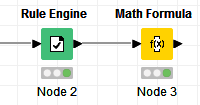Rule Engine:

``````\$Type\$ IN ("personnel expenses", "other operating expenses") => -1
TRUE => 1
``````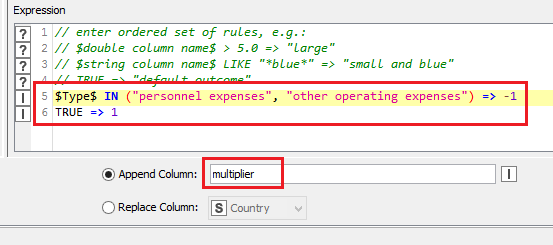Note: Make sure that your Types are written the same way (I just copied and pasted what you wrote in the thread, but your real values in your file are different - it’s not up to me to decide which of the 2 is the correct value and I won’t be diligent about it if you can’t be)

After that, a new column called “multiplier” will be created.

And then in the Math Formula, you can just apply the multiplier to the column Value:
`\$Value\$ * \$multiplier\$`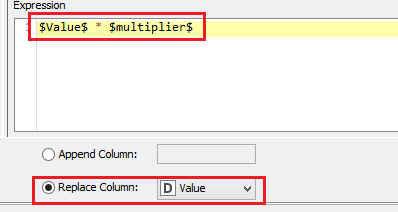Method 2: Column Expressions: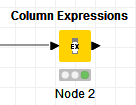``````var lookupArray = arrayCreate("personnel expenses", "other operating expenses")
if(arrayContains(lookupArray, column("Type"))) {
-1 * column("Value")
} else {
column("Value")
}
``````

Note: Again, as with the previous method, make sure that your Types are written the same way